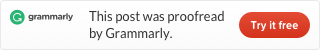Categories

# LeetCode 350 Intersection of Two Arrays II [sort] java

Given two arrays, write a function to compute their intersection.

Example 1:

```Input: nums1 = [1,2,2,1], nums2 = [2,2]
Output: [2,2]```

Example 2:

```Input: nums1 = [4,9,5], nums2 = [9,4,9,8,4]
Output: [4,9]```

Note:

• Each element in the result should appear as many times as it shows in both arrays.
• The result can be in any order.

• What if the given array is already sorted? How would you optimize your algorithm?
• What if nums1‘s size is small compared to nums2‘s size? Which algorithm is better?
• What if elements of nums2 are stored on disk, and the memory is limited such that you cannot load all elements into the memory at once?

We can look at problem 349, these two problems are very similar. The only difference is this problem need to store the count of numbers. We use two hashmaps store the count of the two arrays. Then compare them, select the minimum count number. Code: